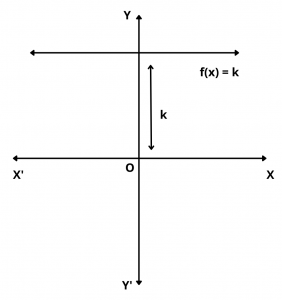# Constant Function – Definition, Graph, Domain and Range

Here you will learn what is constant function with definition, graph and examples.

Let’s begin –

## What is Constant Function ?

Definition : If k is a fixed real number, then a function f(x) given by f(x) = k for all x $$\in$$ R is called a constant function.

For Example, f(x) = 2 , f(x) = 5, f(x) = 2.5 etc.

Sometimes we also call it the constant function k.

## Graph of Constant Function

The graph of a constant function f(x) = k is a straight line parallel to x-axis which is above or below x-axis according as k is positive or negative.

If k = 0, then the straight line is coincident to x-axis.## Domain and Range

We observe that the domain of the constant function f(x) = k  is the set R of all real numbers and range of f is the singleton set {k}.

Domain : R

Range : {k}